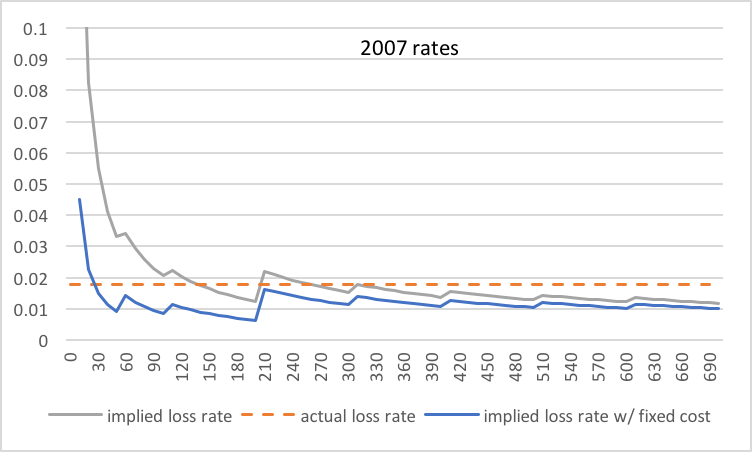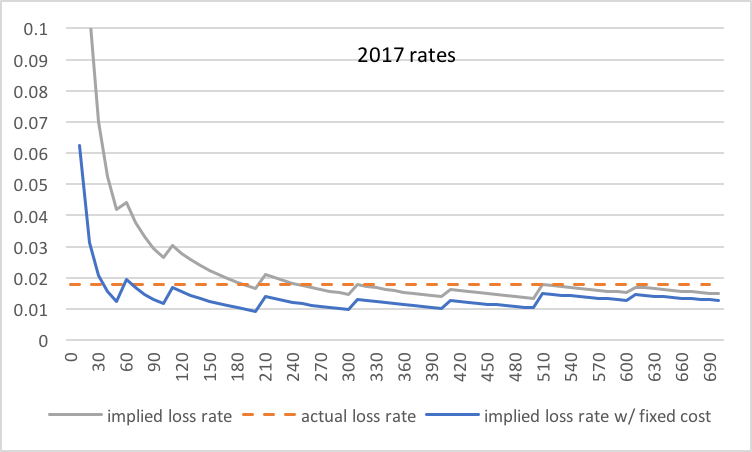### usps insurance rates

What do USPS insurance rates tell us about its operations? Here is a 2007 document regarding insurance rates for domestic mail:

Prices for insurance coverage changed as follows:
Value up to $50 is$1.65.
$50.01 to$100 is $2.05.$100.01 to $200 is$2.45.
$200.01 to$300 is $4.60. The price per additional$100 of insurance, valued over $300 up to$5,000, is $4.60 plus$0.90 per each $100 or fraction thereof. Crudely taking the mid-point of each bracket up to$300, we get implied loss rates of 6.6%, 2.73%, 1.63%, 1.84%, respectively. The rate converges asymptotically to $0.90/$100, or 0.9% implied loss. The numbers have such a wide range that it’s worth taking a closer look.

On page 1 of this 2011-2012 report, it is stated that “lost mail volume is estimated at 1.79 percent.” At these 2007 rates (compare gray and orange lines of below figure), paying for insurance is surely uneconomical for anything under ~$100, but has potentially arbitrageable positive expectation for items valued at ~$300 or more. This is surprising.Interestingly, some of these underpricings have been rectified according to this 2017 document:

Amount for Merchandise Insurance Coverage Desired
$0.01 to$50: $2.10 50.01 to 100: 2.65 100.01 to 200: 3.35 200.01 to 300: 4.40 300.01 to 400: 5.55 400.01 to 500: 6.70 500.01 to 600: 9.15 600.01 to 5,000 (maximum liability is$5,000): $9.15 plus$1.25 per $100 or fraction thereof over$600 in declared value.

These (again, gray and orange lines of below figure) align much more with actual loss rates; still, there is an asymptotic positive expectation for paying for insurance, and in amounts closer to upper bracket limits. Amounts at the lower bracket limits, though, now hug the actual loss rate line, and that may be a part of the pricing decision after all.What about the high rates on low-value items? That’s probably the result of an embedded per-incidence fixed cost. We can back this out by assuming $50 is the target insured amount for the$0 to $50 bracket for the purpose of pricing fixed costs. One model is to obtain an implied loss rate at$50 to match the asymptotic rate, i.e. insurance cost at $50 = asymptotic loss rate *$50 + fixed cost (*), though this can be used (as we do later) at any target insured amount.

At 2007 prices, this gives $1.2 per insured item as the fixed cost portion, or$1.2/0.9% = $133.33 per incidence. Similarly, at 2017 prices, this gives$1.475 per insured item or $1.475/1.25% =$118 per incidence. These costs seem plausible. Implied loss rates adjusted for such costs are plotted as the blue lines in previous figures.

The last oddity is that Express Mail insurance rates have built-in insurance up to $100. Let’s find out how much that is worth. Using the same, older 2007 document that quotes for Express Mail, we have: Prices for Express Mail insurance: The first$100 of value is still provided. Values above $100 are now priced differently than for regular insurance. Value over$100 up to $200 is$0.75.
$200.01 to$500 is $2.10.$500.01 to $5,000 is$2.10 plus $1.35 per each$100 or fraction thereof.

The asymptotic loss rate is priced at 1.35% — interesting that Express Mail should have significantly more implied losses than domestic mail overall. Assuming the same $133.33 per incidence fixed cost as for all domestic mail in 2007, but substituting$100 as the target insured amount into (*), we obtain an insurance cost at $100 of$3.15. So there you go, the “true” price table might be:

$0-$100: $3.15$100-$200:$3.90
$200-$500: $5.25$500-$5000:$5.25 + $1.35 per each additional$100

This is rather favorable for insured values just under the \$500 mark!